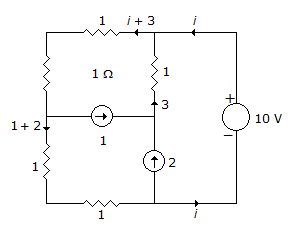# Electronics and Communication Engineering - Exam Questions Papers

31.

A delta modulation system is designed to operate at 3 times the Nyquist rate for a signal with a 3 KHz bandwidth. The quantization step size is 250 mv. The maximum amplitude of a 1 KHz input sinusoidal for which the delta modulator does not show slope overload is :

 A. 716.2 mV B. 500 mV C. 647.87 mV D. 400 mV

Explanation: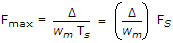.

32.

Values of iL,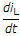, VC and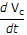at t = 0 are given by,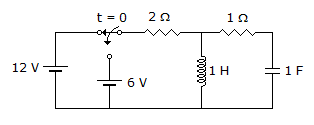A. 4 Amp, - 4 Amp/sec, 12 volts, - 4 volts/s B. 4 Amp, 4 Amp/sec, -12 volts, - 12 volts/s C. 6 Amp, - 2 Amp/sec, 0 volts, - 2 volts/sec D. 6 Amp, 0 Amp/sec, - 2 volts, - 2 volts/sec

Explanation:

For t = 0 +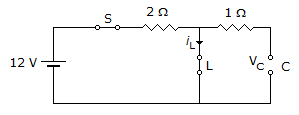iL(0-) =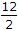= 6 A

VC(0-) = 0 V

For t = 0+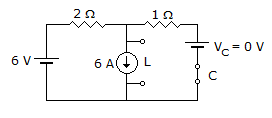iL(0+) = 6

VC(0+) = 0

Apply KCL at node V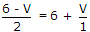6 - V = (6 + v)2

6 - V = 12 + 2V

- 6 = 3V

V = VL = - 2V

At t = 0+,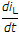= -2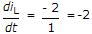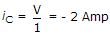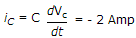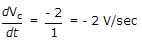iL = 6 Amp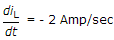VC = 0 V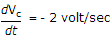.

33.

A continuous time LTI system is described by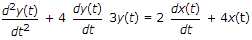Assuming zero initial conditions, the response y(t) of the above system for the input x(t) = e-2t u(t) is given by

 A. (et - e3t) u(t) B. (e-t - 3e-3t) u(t) C. (e-t + e-3t) u(t) D. (et + e3t) u(t)

Explanation: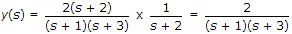y(t) = (e-t - e-3t) u(t).

34.

The analog signal given below is sampled by 600 samples per second
m(t) = 2 sin 480pt + 3 sin 720pt
The folding (maximum) frequency is __________ .

 A. 600 Hz B. 120 Hz C. 300 Hz D. 360 Hz

Explanation:

Folding frequency (max.)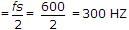.

35.

In the circuit shown, the power supplied by the voltage source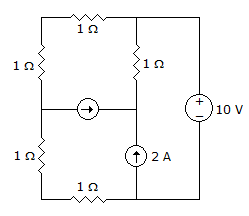A. 0 W B. 5 W C. 10 W D. 100 W

Explanation:

KVL → 10 = 2(i + 3) + 2(i + 2)

10 = 10 + 4ii = 0

Power = 0